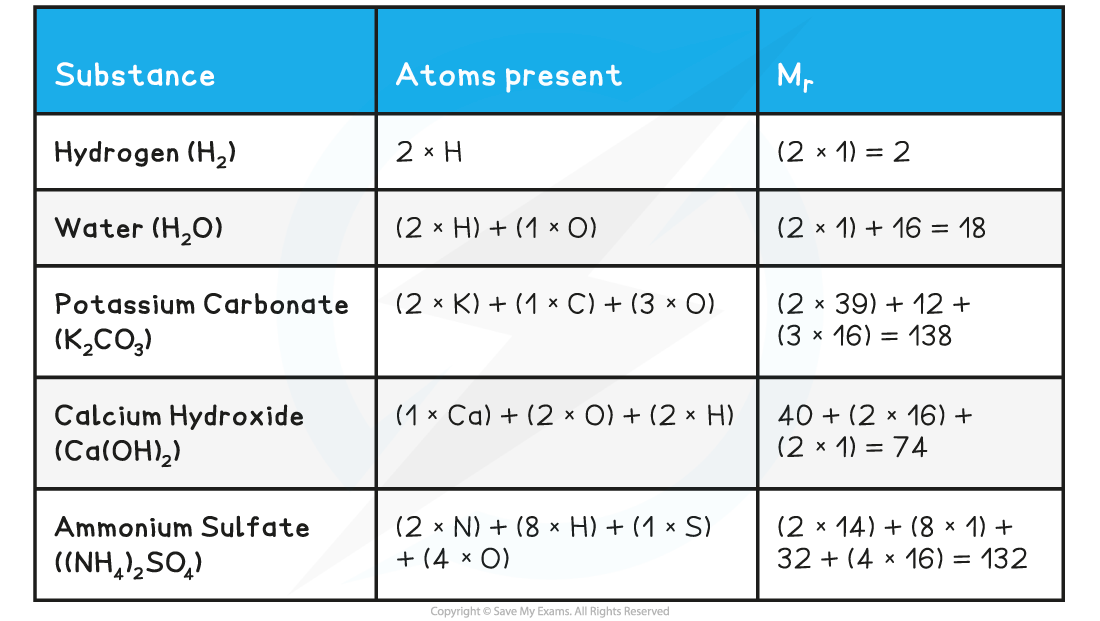# Edexcel A Level Chemistry:复习笔记1.1.3 Relative Mass

### Isotopic & Atomic Mass

• The relative mass of an atom uses the carbon-12 isotope as the international standard
• One atom of carbon-12 has an accepted mass of 1.992646538 x 10-26 kg
• It is not realistic to work with this value so the mass of a carbon-12 atom is fixed as exactly 12 atomic mass units / 12υ
• The standard mass for atomic mass is 1υ
• Therefore, the standard mass for comparison is the mass of 1 ⁄ 12 of a carbon-12 atom

#### Relative isotopic mass

• Relative isotopic mass is defined as the mass of an isotope relative to  1 ⁄ 12  of a carbon-12 atom
• For A Level Chemistry it is common to work with mass values rounded to one decimal place, for example:
• The accurate relative isotopic mass of nitrogen is 14.00307401 but this is rounded to 14.0
• The accurate relative isotopic mass of oxygen is 15.99491464 but this is rounded to 16.0

#### Relative atomic mass

• Most elements on the Periodic Table represent a mixture of different isotopes, which is shown as their relative atomic mass (Ar)
• The relative atomic mass is the weighted mean / average mass of an atom relative to  1 ⁄ 12 of the mass of a carbon-12 atom

### Molecular & Formula Mass

• We have seen previously that the symbol for the relative atomic mass is Ar
• This is calculated from the mass number and relative abundances of all the isotopes of a particular element
• The symbol for the relative formula mass is Mr and it refers to the total mass of the substance
• The term relative formula mass should be used for compounds with giant structures e.g. ionic compounds such as sodium chloride
• If the substance is molecular you can use the term relative molecular mass
• To calculate the Mr of a substance, you have to add up the relative atomic masses of all the atoms present in the formula

Relative Formula Mass Calculations Table#### Exam Tip

It is expected that you will use relative atomic mass values from the Periodic Table

• This means that your values will be more accurate
• e.g. potassium carbonate = (2 x 39.1) + 12.0 + (3 x 16.0) = 138.2

If you are in any doubt whether to use relative molecular mass or relative formula mass, use the latter because it applies to all compounds whether they are ionic or covalent.